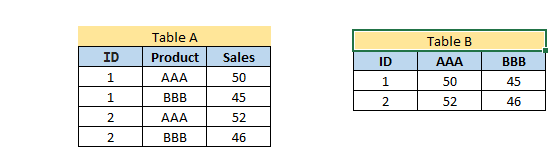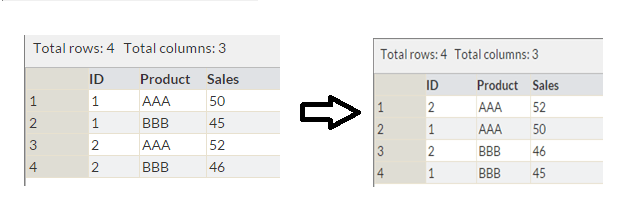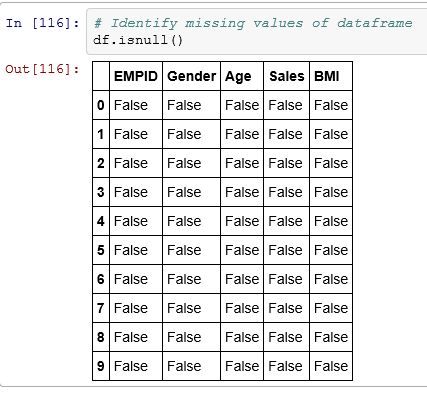Ultimate guide for Data Exploration in Python using NumPy, Matplotlib and Pandas

Introduction

Exploring data sets and developing deep understanding about the data is one of the most important skill every data scientist should possess. People estimate that time spent on these activities can go as high as 80% of the project time in some cases.

Python has been gaining a lot of ground as preferred tool for data scientists lately, and for the right reasons. Ease of learning, powerful libraries with integration of C/C++, production readiness and integration with web stack are some of the main reasons for this move lately.

In this guide, I will use NumPy, Matplotlib, Seaborn and Pandas to perform data exploration. These are powerful libraries to perform data exploration in Python. The idea is to create a ready reference for some of the regular operations required frequently. I am using iPython Notebook to perform data exploration, and would recommend the same for its natural fit for exploratory analysis.

In case you missed it, I would suggest you to refer the baby steps series of Python to understand the basics of python programming.

Contents – Data Exploration

Here are the operation I’ll cover in this article (Refer to this article for similar operations in SAS):

Part 1: How to load data file(s)?

Input data sets can be in various formats (.XLS, .TXT, .CSV, JSON ). In Python, it is easy to load data from any source, due to its simple syntax and availability of predefined libraries. Here I will make use of Pandas. It features a number of functions for reading tabular data as a DataFrame object. Below are the common functions that can be used to read data.Code

import pandas as pd
#Import Library Pandas
df = pd.read_csv("E:/train.csv")  #I am working in Windows environment
#Reading the dataset in a dataframe using Pandas
print df.head(3)  #Print first three observations

OutputCode
df=pd.read_excel("E:/EMP.xlsx", "Data") # Load Data sheet of excel file EMP
Output
print dfCode

df=pd.read_csv(“E:/Test.txt”,sep=’\t’) # Load Data from text file having tab ‘\t’ delimeter print df

Output

Part 2: How to convert a variable to different data type?

Converting a variable data type to other is important and common procedure we perform after loading data. Let’s look at some of the commands to perform these conversions:

• Convert numeric variables to string variables and vice versa

srting_outcome = str(numeric_input) #Converts numeric_input to string_outcome
integer_outcome = int(string_input) #Converts string_input to integer_outcome
float_outcome = float(string_input) #Converts string_input to integer_outcome

The later operations are especially useful when you input value from user using raw_input(). By default, the values are read at string.

• Convert character date to Date:

There are multiple ways to do this. The simplest would be to use datetime library and strptime function. Here is the code:

from datetime import datetime
char_date = 'Apr 1 2015 1:20 PM' #creating example character date
date_obj = datetime.strptime(char_date, '%b %d %Y %I:%M%p')
print date_obj

Part 3: How to transpose a Data set?

Here, I want to transpose Table A into Table B on variable Product. This task can be accomplished by using dataframe.pivot.Code

#Transposing dataframe by a variable

df=pd.read_excel("E:/transpose.xlsx", "Sheet1") # Load Data sheet of excel file EMP
print df
result= df.pivot(index= 'ID', columns='Product', values='Sales')
result

Output

Part 4: How to sort DataFrame?

Sorting of data can be done using dataframe.sort(). It can be based on multiple variables and ascending or descending both order.

Code

#Sorting Dataframe
df=pd.read_excel("E:/transpose.xlsx", "Sheet1") #Add by variable name(s) to sort
print df.sort(['Product','Sales'], ascending=[True, False])Above, we have a table with variables ID, Product and Sales. Now, we want to sort it by Product and Sales (in descending order) as shown in table 2.

Part 5: How to create plots (Histogram, Scatter, Box Plot)?Data visualization always helps to understand the data easily. Python has library like matplotlib and seaborn to create multiple graphs effectively. Let’s look at the some of the visualization to understand below behavior of variable(s) .

• The distribution of age
• Relation between age and sales; and
• If sales are normally distributed or not?

Histogram:

Code

#Plot Histogram
import matplotlib.pyplot as plt
import pandas as pd
#Plots in matplotlib reside within a figure object, use plt.figure to create new figure
fig=plt.figure()
#Create one or more subplots using add_subplot, because you can't create blank figure
#Variable
ax.hist(df['Age'],bins = 5)
#Labels and Tit
plt.title('Age distribution')
plt.xlabel('Age')
plt.ylabel('#Employee')
plt.show()

OutputScatter plot:

Code

#Plots in matplotlib reside within a figure object, use plt.figure to create new figure fig=plt.figure()
#Create one or more subplots using add_subplot, because you can't create blank figure
#Variable
ax.scatter(df['Age'],df['Sales'])
#Labels and Tit
plt.title('Sales and Age distribution')
plt.xlabel('Age')
plt.ylabel('Sales')
plt.show()

OutputBox-plot:

Code

import seaborn as sns
sns.boxplot(df['Age'])
sns.despine()

Output

Part 6: How to generate frequency tables with pandas?

Frequency Tables can be used to understand the distribution of a categorical variable or n categorical variables using frequency tables.

Code

import pandas as pd
print df
test= df.groupby(['Gender','BMI'])
test.size()

Output

Part 7: How to do sample Data set in Python?

To select sample of a data set, we will use library numpy and random. Sampling of data set always helps to understand data quickly.

Let’s say, from EMP table, I want to select random sample of 5 employee.

Code

#Create Sample dataframe
import numpy as np
import pandas as pd
from random import sample
# create random index
rindex = np.array(sample(xrange(len(df)), 5))
# get 5 random rows from df
dfr = df.ix[rindex]
print dfr

Output

Part 8: How to remove duplicate values of a variable?

Often, we encounter duplicate observations. To tackle this in python, we can use dataframe.drop_duplicates().

Code

#Remove Duplicate Values based on values of variables "Gender" and "BMI"
rem_dup=df.drop_duplicates(['Gender', 'BMI'])
print rem_dup

OutputPart 9: How to group variables in Python to calculate count, average, sum?

To understand the count, average and sum of variable, I would suggest you to use dataframe.describe() with groupby().

Let’s look at the code:

Code

test= df.groupby(['Gender'])
test.describe()

Output

Part 10: How to recognize and Treat missing values and outliers?

To identify missing values , we can use dataframe.isnull(). You can also refer article “Data Munging in Python (using Pandas)“, here we have done a case study to recognize and treat missing and outlier values.

Code

# Identify missing values of dataframe
df.isnull()

OutputTo treat missing values, there are various imputation methods available. You can refer these articles for methods to detect Outlier and Missing values. Imputation methods for both missing and outlier values are almost similar. Here we will discuss general case imputation methods to replace missing values. Let’s do it using an example:

Code:

#Example to impute missing values in Age by the mean
import numpy as np
meanAge = np.mean(df.Age)     #Using numpy mean function to calculate the mean value
df.Age = df.Age.fillna(meanAge) #replacing missing values in the DataFrame

Part 11: How to merge / join data sets?

Joining / merging is one of the common operation required to integrate datasets from different sources. They can be handled effectively in Pandas using merge:

Code:

df_new = pd.merge(df1, df2, how = 'inner', left_index = True, right_index = True) # merges df1 and df2 on index
# By changing how = 'outer', you can do outer join.
# Similarly how = 'left' will do a left join
# You can also specify the columns to join instead of indexes, which are used by default.

End Notes:

In this comprehensive guide, we looked at the Python codes for various steps in data exploration and munging. We also looked at the python libraries like Pandas, Numpy, Matplotlib and Seaborn to perform these steps. In next article, I will reveal the codes to perform these steps in R.

Also See: If you have any doubts pertaining to Python, feel free to discuss with us.

Did you find the article useful? Do let us know your thoughts about this guide in the comments section below.

If you like what you just read & want to continue your analytics learning, subscribe to our emails, follow us on twitter or like our facebook page.

You can also read this article on Analytics Vidhya's Android APPThis article is quite old and you might not get a prompt response from the author. We request you to post this comment on Analytics Vidhya's Discussion portal to get your queries resolved

•Vikas says:

Need the same thing/article in R. Please do need full asap.
Thanks.

•Sunil Ray says:

Hi Vikas,

Guide for Data Exploration using R is live now. You can access it here http://www.analyticsvidhya.com/blog/2015/04/comprehensive-guide-data-exploration-r/

Thanks

•brianpiercy says:

Awesome list. Thanks so much!

•Sandeep Karkhanis says:

I’m struggling to find methods (read packages / libraries) to carry out imputation in Python beyond the usual mean/ median approach…coming from an R background , there are tonnes of good packages to carry out very sophisticated imputation…this is something I miss in Python (it may be my lack of knowledge).

i tried r2py to call imputation packages from R in python but it doesnt run as smoothly

do you have suggestions in this regards?

•Ken Stulce says:

Fantastic article with great examples! Thank you.

•subbrao says:

Great article. I learned something today.
Any books which helps me to do these kind of data analysis?

•K CHENDRA TEJA says:

good article

•venugopal says:

super

•Animesh says:

•Sunny says:

Thank you so much. This was very helpful. 🙂

•Ratna Kishore Jupudi says:

Nice summary Thank you.

•Ashok Bharat says:

Very Useful, covered all important data manipulation/exploration libraries, very handy.. !

•anubhaw says:

AttributeError: ‘DataFrame’ object has no attribute ‘sort’

•Aishwarya Singh says:

Hi Anubhaw,Enroll NowEnroll Now